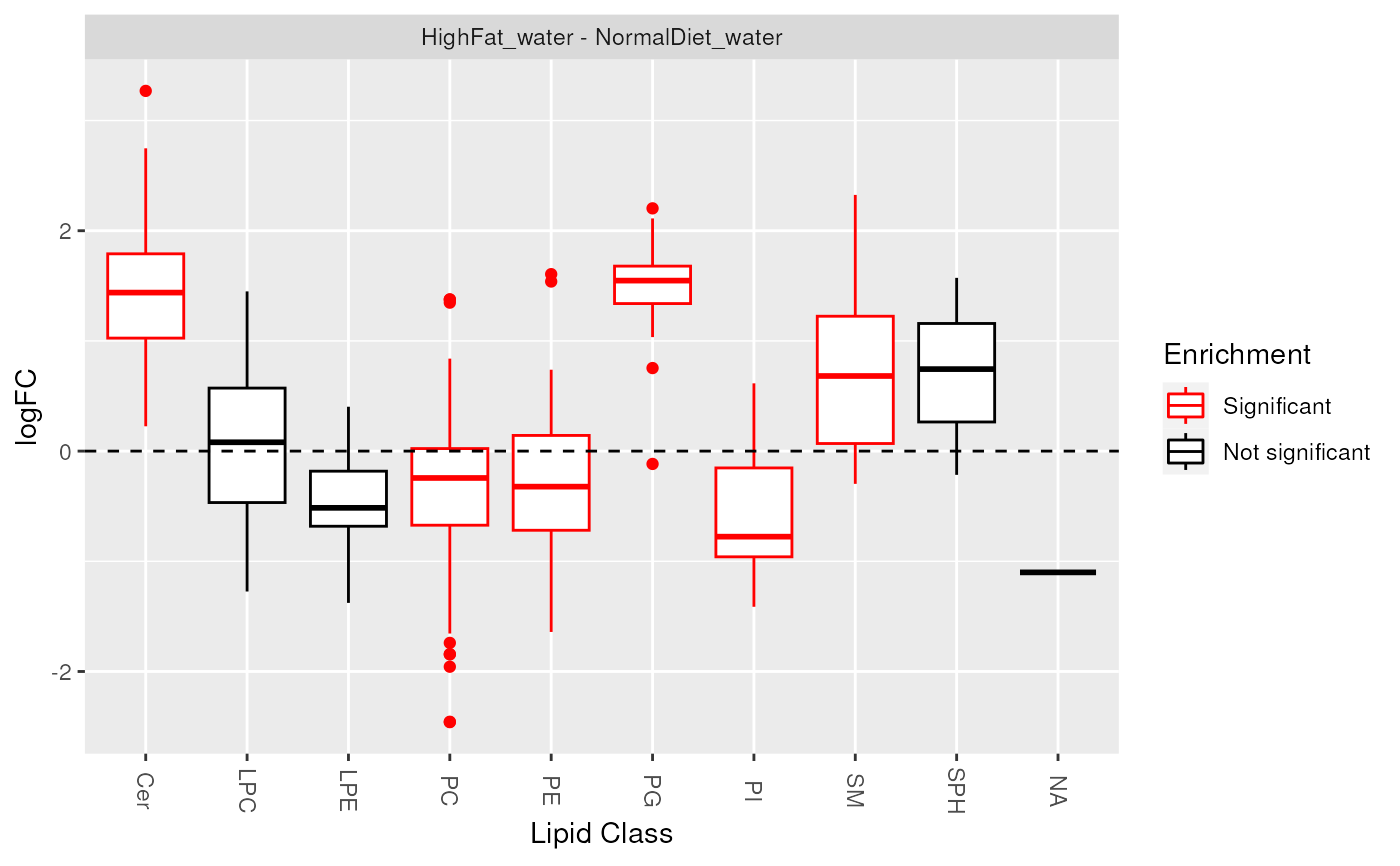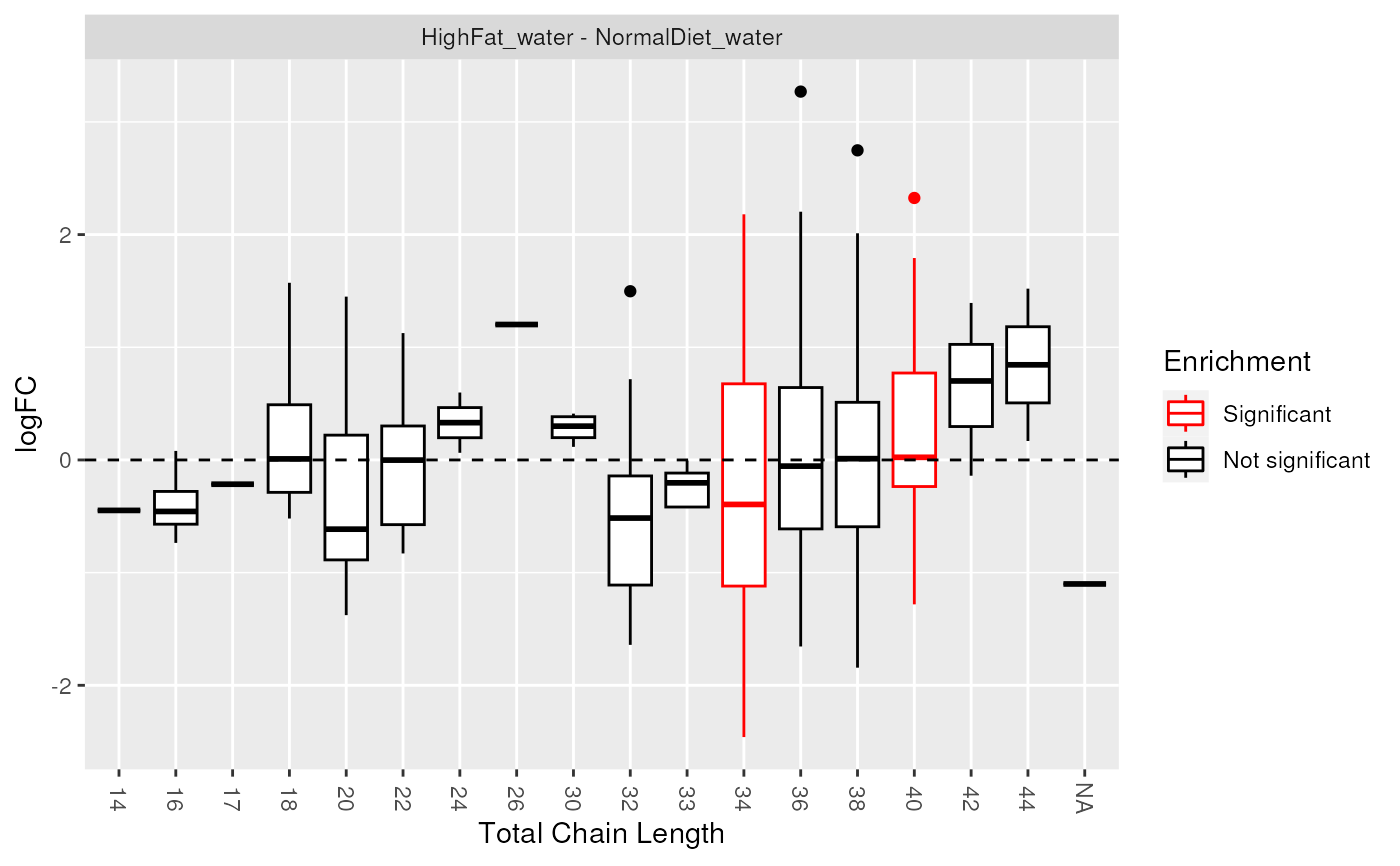Lipid set enrichment analysis (LSEA)

lsea(
de.results,
rank.by = c("logFC", "P.Value", "adj.P.Val"),
min_size = 2,
...
)

significant_lipidsets(enrich.results, p.cutoff = 0.05, size.cutoff = 2)

plot_class_enrichment(de.results, significant.sets, measure = "logFC")

plot_enrichment(
de.results,
significant.sets,
annotation = c("class", "length", "unsat"),
measure = "logFC"
)

## Arguments

de.results

Output of de_analysis().

rank.by

Statistic used to rank the lipid list. Default is logFC.

min_size

Minimum number of molecules in a set to be included in enrichment.

...

Extra parameters passed to fgsea::fgsea().

enrich.results

Output of lsea().

p.cutoff

Significance threshold. Default is 0.05.

size.cutoff

Minimum number of lipids in a set tested for enrichment. Default is 2.

significant.sets

List of significantly changed lipid sets (output of significant_lipidsets()).

measure

Which measure to plot the distribution of: logFC, P.Value, Adj.P.Val. Default is logFC.

annotation

Which lipid set collection to plot.

## Value

lsea returns enrichment results (data.frame) as returned from fgsea::fgsea(). The results also contain the following attributes:

• de.results Original de.results input.

• rank.by Measure used to rank lipid molecules.

• sets Lipid sets tested, with their member molecules.

significant_lipidsets returns a list of character vectors of significantly enriched sets for each contrast.

plot_enrichment returns a ggplot object.

## Functions

• significant_lipidsets: gets a list of significantly changed lipid sets

• plot_enrichment: is usually used to look at log2 fold change distribution of lipids in each class, chain length or unsaturation, marking significantly enriched sets. It can also be used to plot P.Value or Adj.P.Val.

## Examples

data(data_normalized)
de_results <- de_analysis(
data_normalized,
HighFat_water - NormalDiet_water,
measure = "Area"
)
enrich_results <- lsea(
de_results,
rank.by = "logFC", min_size = 4, nperm = 1000
)
#> Warning: There are ties in the preranked stats (5.78% of the list).
#> The order of those tied genes will be arbitrary, which may produce unexpected results.
sig_lipidsets <- significant_lipidsets(enrich_results)
plot_enrichment(de_results, sig_lipidsets, annotation="class")plot_enrichment(de_results, sig_lipidsets, annotation="length")